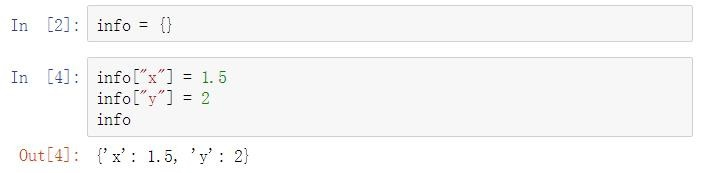﻿ python修改字典键（key）的方法_python_脚本之家
python# python修改字典键（key）的方法

python字典中，值可任意更改；但键是唯一的，不支持直接修改。若真的需要修改字典中的键，可通过几种间接方式实现。

`info = {}````info["x"] = 1.5

info["y"] = 2

info
``````info = {"x":1.5 ,"y":2}

info["z"] = info("x")

print(info)
``````info = {"x":1.5 ,"y":2}

info["z"] = info.pop("x")

info
``````info = {"x":1.5 ,"y":2}

info.update({"z":info.pop("x")})

info
``````info = {"x":1.5 ,"y":2}

info["z"] = info["x"]

del info["x"]

info
``````dict={'a':1, 'b':2}

dict["c"] = dict.pop("a")
```

```dict={'a':1, 'b':2}

dict.update({'c':dict.pop("a")})
```

```dict={'a':1, 'b':2}

dict['c']=dict['a']

del dict['a']
```